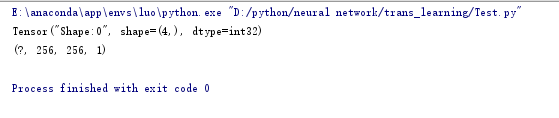``````import tensorflow as tf

x = tf.placeholder(shape=[None, 256, 256, 1], dtype=tf.float32)
print(tf.shape(x))
print(x.get_shape())``````x.get_shape()得到的是元組表示的shape，包含每一個維度